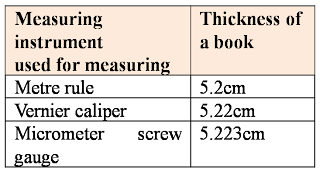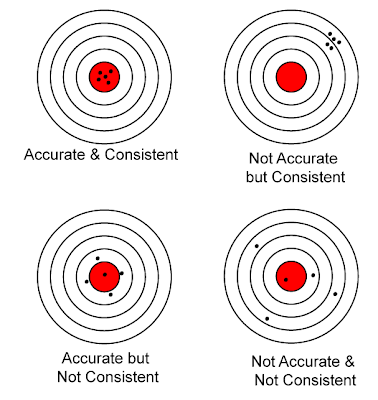# Consistency, Accuracy and Sensitivity

### Precision

1. Precision is the ability of an instrument in measuring a quantity in a consistent manner with only a small relative deviation between readings.
2. The precision of a reading can be indicated by its relative deviation.
3. The relative deviation is the percentage of mean deviation for a set of measurements and it is defined by the following formula:### Accuracy

1. The accuracy of a measurement is the approximation of the measurement to the actual value for a certain quantity of Physics.
2. The measurement is more accurate if its number of significant figures increases.
3. Table above shows that the micrometer screw gauge is more accurate than the other measuring instruments.
4.5. The accuracy of a measurement can be increased by
1. taking a number of repeat readings to calculate the mean value of the reading.
2. avoiding the end errors or zero errors.
3. taking into account the zero and parallax errors.
4. using more sensitive equipment such as a vernier caliper to replace a ruler.
6. The difference between precision and accuracy can be shown by the spread of shooting of a target (as shown in Diagram below).### Sensitivity

1. The sensitivity of an instrument is its ability to detect small changes in the quantity that is being measured.
2. Thus, a sensitive instrument can quickly detect a small change in measurement.
3. Measuring instruments that have smaller scale parts are more sensitive.
4. Sensitive instruments need not necessarily be accurate.